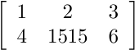## Multiplying dense matrices with large integer coefficients

### Problem specification

The problem is to compute as quickly as possible the product of two square integer matrices. The size of an instance is depending on both the matrix order, and the bit size of the coefficients in the matrix.
The participants are free to use any piece of software for integer arithmetic (e.g. The GMP library or MPIR) and matrix computations (e.g. LinBox, FFLAS-FFPACK) or any other library of their choice.

### Specification of the program input/output

The participant must provide a program with the following specification:
• Matrix file storage format: a m×n matrix will be stored in a 1 + m×n lines file:
• First line: nrows ncols
• Each of the following lines will contain one coefficient. They are read from the matrix following the row-major order: line ((i - 1)×ncols + j) + 1 contains the coefficient (i, j) of the matrix (indices starting at 1).
Example: the matrixwill be stored in a file as
2 3
1
2
3
4
1515
6
• Usage: To compute the produte C = A × B:
matmul A B C
where the arguments A, B and C are files storing matrices

### Reference implementation

We provide a naive sequential implementation using GMP: intmatmul.cpp.

### Library of instances

For each of the following instances, sorted by increasing difficulty, we provide the two matrices A and B. The challenge is to compute the product A × B. n is the matrix dimension and b is the size in bits of the largest coefficient.
• Instance 1: n=100, b=10000 A, B
• Instance 2: n=1000, b=200 A, B
• Instance 3: n=10000, b=8,60 A, B
• Instance 4: n=7000, b=50 A, B